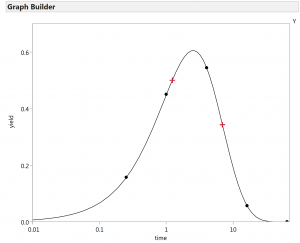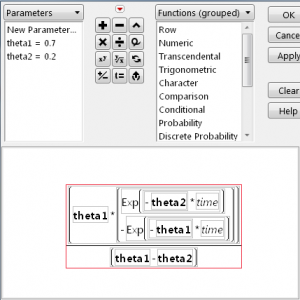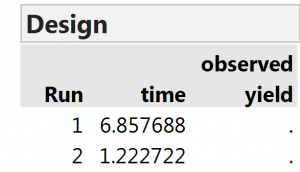Box & Lucas: Designed experiments for nonlinear models

All this month, I'm writing about George E.P. Box, as part of the celebration of the International Year of Statistics. Last week, I wrote about Box-Behnken designs for fitting response surface models. In this post, I want to tell you about the paper Box wrote in 1959 with H. L. “Curly” Lucas.

I was interested in this paper for several reasons. First, Lucas is the father of one of my colleagues at SAS, Bob Lucas. I was just talking to Bob about this blog post, and he told me that his father was called Curly not because he had curls but because he looked like Curly from the Three Stooges. I was also interested in this paper because it is one of the first papers on how to design experiments for models that are nonlinear in the parameters.

How does the paper begin?

The paper starts with an example of the kind of problem the authors want to address. They imagine a chemical reaction where a substance A decomposes to form substance B, which in turn decomposes to form substance C. The yield, y, of B as a function of time, t, is

y = θ 1 { exp (θ 2 t )- exp( θ 1 t)}/ ( θ 1 - θ 2 )

The problem is to choose reaction times at which to observe the yield in order to get the most precise estimates possible of the unknown parameters, θ 1 and θ 2 .

What happens next?

A short subsection then deals with the practicalities of choosing ranges for the factors. For example, in the reaction example, the time must be positive. The following section contains the main analytical development. Here, Box and Lucas introduce what amounts to a local D-optimality criterion for the nonlinear model. They point out that the information in the design depends on the unknown values of the parameters, so you need an initial guess at the parameters to get started.

They also suggest two ideas for further work that seem prescient. One idea was to consider a prior distribution on the unknown parameters. This naturally leads to a Bayesian D-optimality criterion, which is what the JMP nonlinear designer uses.

Their second idea was to consider a sequential approach whereby the researcher augments the current data by adding points that are optimal with respect to the current parameter estimates. Then, new responses are acquired, and the model is updated. Assuming the fitted parameters change, there would be another set of optimal runs to do. This is current practice in some dose response modeling studies.

At this point, they return to their motivating example, making guesses of that θ 1 = 0.7 and θ 2 = 0.2. Given these parameter values, they plot the yield as a function of time.

Figure 1 shows the JMP Graph Builder plot of their function. The two red plus signs are at the optimal two times for getting precise estimates of the parameters given that the initial guesses are close to being correct.Figure 2 shows the way to enter a function for use with the nonlinear designer in JMP.Table 1 shows the locally optimal design produced by JMP using initial parameter values of 0.7 and 0.2.In the paper, Box and Lucas report the two optimal times as 1.23 and 6.86. Pretty close for 1959!

How does the paper end?

The rest of the paper deals with applying there methods to other examples with one or two unknown parameters. In their discussion at the end of the article, they make one more really interesting point. Sometimes, the parameters enter the model in such a way that even the best design is incapable of producing precise parameter estimates. They point out that in many cases, the predicted responses are still good even if the parameters are not nailed down due to high correlations among them. From there, it would only take a small step to suggest an optimality criterion that minimizes the average variance of the responses.

G. E. P. Box and H. L. Lucas (1959) “Design of Experiments in Non-Linear Situations” Biometrika Vol. 46 No. 1 pp. 77-80.